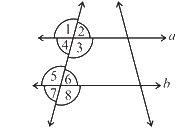# Ex.5.2 Q1 Lines and Angles - NCERT Maths Class 7

Go back to  'Ex.5.2'

## Question

State the property that is used in each of the following statements?

(i)   If $$a || b,$$ then $$\angle1 = \angle5.$$

(ii)  If $$\angle4 = \angle6,$$ then $$a || b.$$

(iii) If $$\angle4 + \angle5 = 180^\circ,$$ then $$a || b.$$Video Solution
Lines & Angles
Ex 5.2 | Question 1

## Text Solution

Steps:

(i) If two parallel lines are intersected by a transversal, then each pair of corresponding angles are equal .

(ii) When a transversal intersects two parallel lines such that if pair of alternate interior angles are equal then the lines are parallel.

(iii) When a transversal intersects two parallel line such that pair of interior angles on the same side of transversal are supplementary, then the lines are parallel.

Learn from the best math teachers and top your exams

• Live one on one classroom and doubt clearing
• Practice worksheets in and after class for conceptual clarity
• Personalized curriculum to keep up with school